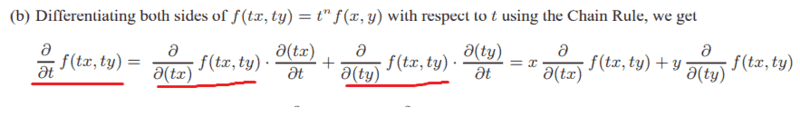# Not understanding these manipulations involving Partial Derivatives

MatinSAR
Homework Statement:
Find partial derivatives
Relevant Equations:
dy/dx=(dy/dt)(dt/dx)ergospherical
It's differentiating ##f## with respect to its arguments, then differentiating the arguments with respect to ##t##. It might be clearer if you write ##u = tx## and ##v=ty##, then
$$\partial f(u,v) / \partial t = (\partial f/ \partial u) (\partial u/ \partial t) + (\partial f/ \partial v) (\partial v/ \partial t)$$

•SammyS, Mark44 and MatinSAR
MatinSAR
It's differentiating ##f## with respect to its arguments, then differentiating the arguments with respect to ##t##. It might be clearer if you write ##u = tx## and ##v=ty##, then
$$\partial f(u,v) / \partial t = (\partial f/ \partial u) (\partial u/ \partial t) + (\partial f/ \partial v) (\partial v/ \partial t)$$
That "tx" confused me ...
Now it's clear...
Thank you for your time 🙏🙏

•berkeman